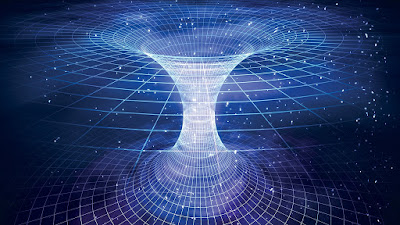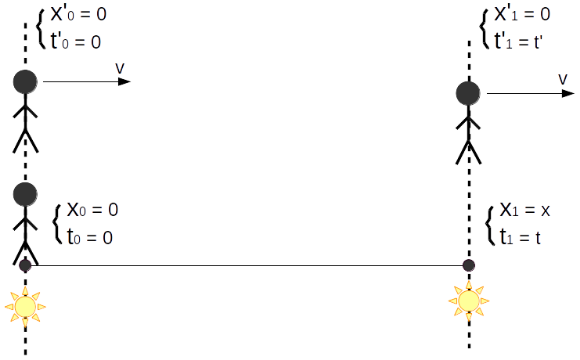The speed of light always is ‘c’, no matter where we are (either on the moving body or on any rest ‘ground’). This is one of the two fundamental assumptions based on which Einstein’s special theory of relativity stands. This is, as a matter of fact, the result of experiment, not imagination or assumption of Einstein, Feynman, or anybody else. The famous experiment is called Michelson-Morley experiment, the details of which can be found in Wikipedia. The basic idea of this experiment is: assuming the speed of light ‘c’ is specifically relative to something (historically, the ‘something’ here was called aether, from what I remember), then we know our earth is rotating around the self-axis, thus the speed of light, of course, is NOT ‘c’, if our previous assumption (‘c’ is specifically relative to aether) stands. Then using the facility designed by Michelson and Morley, we should observe some corresponding effect, which is the result of the changing of speed of light relative to our earth. I won’t bother with what the effect will be like (detailed information can be found in the link at the bottom), but I can tell the result: no expected effect was observed if our previous assumption stands! That’s it, it seems that we have to accept the experimental FACT that speed of light is always ‘c’ – relative to the observer on spacecraft, or relative to our earth, or relative to anything moving on the earth, or, or, relative to ANYTHING in the universe. Actually, it was just the result of the famous Michelson-Morley experiment (and some other following experiments – see the link below) that directly leads to the invention of the special theory of relativity. Moreover, the invariance of speed of light is one of the two basic principles of special theory of relativity. Furthermore, when we are talking about TIME, we actually mean the interval (along time axis) between two EVENTS. So imagine the process of a ball rising up from the ground and falling back to the ground. So the two events, in this case, are ‘ball leaves the surface – starting to rise up’ and ‘ball comes back to the surface – finishing falling down’. Then two observers – one moves together with the ball and the other one stands on the rest ‘ground’ – observe exactly the same two events, are the time (interval) measured by these two guys going to be different? The answer is: Yes. Why? Why? Why? Well, again, this is what we have to accept as the FACT. However, it’s worth a bit explanation, and now we need to go back to the experimental FACT – the speed of light does not change from frame to frame (ignoring the influence of medium, e.g. from air to water, and all the other effects). It is based on this FACT that people (Lorentz should be one of them, I think) obtained the invariant quantity called space-time interval for ANY frames. The definition is,

${s^2} = - {c^2}{(\Delta t)^2} + {(\Delta x)^2} + {(\Delta y)^2} + {(\Delta z)^2}$

To better express the idea, here I give an example as following,Time and length by different observer.

In the figure, there are two observers in one-dimension frames – one is standing on the ground, and the other is moving relative to the ground with the speed of v. Let’s call the axis x-axis, and the frame for observer-1 and observer-2 is named frame-1 (for which we use the notion x’ and t’) and frame-2 (for which we use the notion of x’’ and t’’), respectively. Moreover, both observer-1 and observer-2 stays at their own origins. Now, let’s imagine two bulb emitting light (as shown in the figure) one after another. Right at the beginning, bulb-1 emits light at the origin of frame-1, and just at that moment, observer-2 is also at the origin of frame-1 (which means the origins of frame-1 and frame-2 coincides with each other). Then we record the position and time for the event of bulb-1 emitting light, in two frames. For frame-1, it is: ({x_0} = 0), ({t_0} = 0), and for frame-2, it is: x’ = 0, t’ = 0. Then after some moments, observer-2 (remember? observer-2 is moving with speed of v, in frame-1, or as seen from observer-1) arrives at the position where bulb-2 is placed in frame-1, and JUST AT THAT EXACT MOMENT, bulb-2 emits light. Then we write down the position and time for the event of bulb-2 emitting light. For frame-1, it is: ({x_1} = x), ({t_1} = t), and for frame-2, it is: ({x’_1} = 0), ({t’_1} = t). You guess what? The two events happens at the same place as seen by observer-2 (or we say, in frame-2)!
So now, we have two events - emission of the two bulbs. What we suspect is: is t (the time interval for these two events observed in frame-1, or by observer-1) and t’ (the time interval for these two events observed in frame-2, or by observer-2), the same? Or different? Let’s have a look. As is already given above, we have the invariant quantity s from frame to frame, thus we have,

$- {c^2}{t^2} + {x^2} = - {c^2}t{'^2} + 0$

What else do we have? Remember observer-2 arrives at bulb-2 at the exact moment when bulb-2 emits light? So definitely we should have,

$vt = x$

By replacing $$x$$ into the previous equation, we have,

$- {c^2}{t^2} + {v^2}{t^2} = - {c^2}t{'^2}$

Rearranging the above equation, it is easy to get,

$t = \frac{1}{\sqrt{1 - \frac{v^2}{c^2}}}t' = \gamma t'$

So, so, so, finally, without assuming anything except accepting the FACT that speed of light doesn’t change from frame to frame (based on which we then have the invariant quantity space-time interval), we obtain the result that for the two events that we cannot visually ‘feel’ any difference when changing the observing frame, the time interval observed in two different frames (one is moving relative to another), is indeed different! – They are linked up by the so called γ factor, as you can see from the above formula.
What else can we say? Well, I should say, the difference of time interval observed in different frames (moving relative to each other) between two events, is some kind of PROPERTY of our space, and time. I am afraid, we have to accept it, maybe, no other choice.

N. B. The original post is from my answer to people’s question concerning the special theory of relativity on Stack Exchange. Click me to go to Stack Exchange Q & A.

References